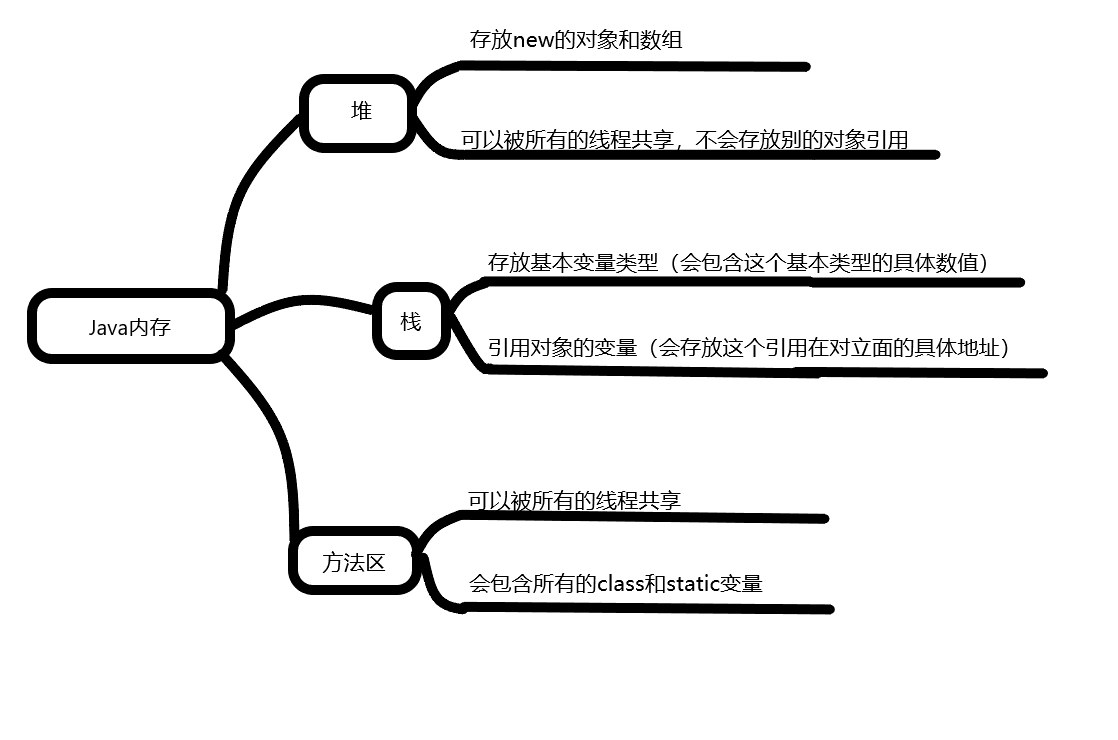# 数组

## 数组的定义

• 数组是相同类型数据的有序集合。
• 数组描述的是相同类型的若干个数据，按照一定的先后次序排列组合而成。
• 其中，每一个数据称作一个数组元素，每个数组元素元素可以通过一个下标来访问他们。

## 数组声明创建

• 首先必须声明数组变量，才能在程序中使用数组。下面是声明数组变量的语法：

dataType[] arrayRefVar; //首选方法
或
dataType arrayRefVar[]; //效果相同，但不是首选方法

• Java语言使用new操作符来创建数组，语法如下：

dataType[] arrayRefVar = new dataType[arraySize];

//声明，创建和使用数组
int numes[] = new int；//声明创建一个长度为10的数组
nums=1;
nums=2;
nums=3;
nums=4;
nums=5;
nums=6;
nums=7;
nums=8;
nums=9;
nums=10;
int sum = 0;
//arrays.length 数组的长度。
for(int i = 0;i<=nums.length;i++){
sum += nums[i];
}
System.out.println(sum);


## 内存分析## 三种初始化

• 静态初始化

int[] a = {1,2,3};
Man[] mans = {new Man(1,1),new Man(2,2)};

• 动态初始化

int[] a = new int;
a = 1;
a = 2;

• 数组的默认初始化

数组是引用类型，他的元素相当于类的实例变量，因此数组一经分配空间，其中的每个元素也被按照实例变量的方式被隐式初始化。

## 数组的四个基本特点

• 其长度是确定的。数组一旦被创建，它的大小就是不可以改变的。
• 其元素必须是相同类型，不允许出现混合类型。
• 数组中的元素可以是任何数据类型，包括基本类型和引用类型。
• 数组变量属于引用类型，数组也可以看成是对象，数组中的每个元素相当于该对象的成员变量。数组本身就是对象，Java中对象是在堆中的，因此数组无论保存原始类型还是其他对象类型，数组对象本身就是在堆中的。

## 数组边界

• 下标的合法区间：[0，length-1]，如果越界就会报错；

public static void main(String[] args){
int[] s = new int ;
System.out.println(a);
}

• ArrayIndexOutOfBoundsException:数组下标越界异常！

• 小结：

• 数组也是相同数据类型(数据类型可以为任意类型)的有序集合
• 数组也是对象。数组元素相当于对象的成员变量
• 数组长度是确定的，不可变的。

## 数组的使用

• 普通的For循环

• For-Each循环(增强For循环)

int arrays[]={1,2,3,4,5}
for(int arr:arrays){
//for(元素类型 元素名称:遍历数组(集合))
System.out.println(arr)
}
//多用于打印

• 数组作方法入参

public static void printArray(int arrays[]){
for(int i = 0;i<arrays.length;i++){
System.out.print(arrays[i]+" ");
}
}
public static void main(String[] args){
int arrays[] = {1,2,3,4,5}
printArray(arrays)
}

• 数组作返回值

public static void printArray(int arrays[]){
for(int i = 0;i<arrays.length;i++){
System.out.print(arrays[i]+" ");
}
}
//反转数组
public static int[] reverse(int[] arrays){
int[] result = new int[arrays.length];
//反转的操作
for (int i=0,j=result.length-;i<arrays.length;i++,j--){
//result[] = arrays[i];
result[j] = arrays[i];
}
public static void main(String[] args) {
int arrays[] = {1,2,3,4,5};
int reverse[] = reverse(arrays);
printArray(reverse);
}



## 多维数组

• 多维数组可以看成是数组的数组，比如二维数组就是一个特殊的一组数组，其每一个元素都是一个一堆数组

• 二维数组

int a[][] = new int

• 解析：以上二维数组a可以看成一个两行五列的数组。

• 思考：多维数组的使用？

        /*
1,2 array array
2,3 array array
3,4 array array
4,5 array array
*/
int[][] array = {{1, 2}, {2, 3}, {3, 4}, {4, 5}};
System.out.println(array);
for (int i = 0;i<array.length;i++){
for (int j = 0;j<array[i].length;j++){
System.out.println(array[i][j]);
}
}


## Arrays类

• 数组的工具类java.util.Arrays

• 具有以下常用功能：

• 给数组赋值：通过fill方法。

• 给数组排序：通过sort方法，按升序。

• 比较数组：通过equals方法比较数组中元素值是否相等。

• 查找数组元素：通过binarySearch方法能对排序好的数组进行二分查找法操作。

import java.util.Arrays;
public class ArrayDemo {
public static void main(String[] args) {
int [] a = {5144,895614,8525244,65231,20};
//打印数组元素Arrays.toString
System.out.println(Arrays.toString(a));
//排序
Arrays.sort(a);
System.out.println(Arrays.toString(a));
//赋值
Arrays.fill(a,2,4,0);
System.out.println(Arrays.toString(a));
}
}



## 冒泡排序

import java.util.Arrays;

public class ArrayDemo {
public static void main(String[] args){
int[] a = {17,2,90,85,65,100};
int[] sort = sort(a);
System.out.println(Arrays.toString(sort));
}

//冒泡排序
//1.比较数组中，两个相邻的元素，如果第一个数比第二个数大，我们就交换他们的位置
//2.每一次比较，都会产生出一个最大或者最小的数字
//3.下一轮则可以少一次排序
//4.依次循环，直到结束

public static int[] sort(int[] array){
//临时变量
int temp = 0;

//外层循环，判断我们这个循环要走多少次
for (int i = 0;i<array.length-1;i++){
//内层循环，比较判断两个数，如果第一个数比第二个数大，则交换位置
for (int j = 0;j<array.length-1-i;j++){
if (array[j]>array[j+1]){
temp = array[j];
array[j] = array[j+1];
array[j+1] = temp;
a = true;
}
}
}
return array;

}
}



## 稀疏数组

• 当一个数组中大部分元素为0，或者为同一值的数组时，可以使用稀疏数组来保存该数组。(相当于一个记录行，列，值的列表)
• 稀疏数组的处理方式是：
• 记录数组一共有几行几列，有多少个不同值
• 把具有不通知的元素和行列及值记录在一个小规模的数组中，从而缩小程序的规模
public class ArrayDemo {
public static void main(String[] args) {
//1.创建一个二维数组 例：一个五子棋盘11*11 0代表无棋子，1代表黑棋，2代表白棋
int[][] array1 = new int;
array1 = 1;
array1 = 2;
//输出原始的数组
System.out.println("输出原始的数组");
for (int arr[]:array1){
for (int i : arr) {
System.out.print(i+"\t");

}
System.out.println();
}

//转换为稀疏数组保存
//1.获取有效值的个数
int num = 0;
for (int i = 0;i<11;i++){
for (int j = 0;j<11;j++){
if (array1[i][j]!=0){
num++;
}
}
}
System.out.println("有效值的个数"+num);

//创建一个数组
int[][] array2 = new int[num+1];
array2  = 11;//11行
array2  = 11;//11列
array2  = num;//有num个值

//遍历二维数组，讲非零的值存放到稀疏数组里面
int count = 0;
for (int i = 0;i<array1.length;i++){
for (int j = 0;j<array1[i].length;j++){
if (array1[i][j]!=0){
count++;
array2[count] = i;
array2[count] = j;
array2[count] = array1[i][j];
}
}
}
//输出稀疏数组
System.out.println("稀疏数组");
for (int i = 0;i<array2.length;i++){
System.out.println(array2[i]+"\t"+array2[i]+"\t"+array2[i]);
}
System.out.println("还原");
//1.读取稀疏数组
int[][] array3 = new int[array2][array2];
//2.给其中的元素还原它的值
for (int i = 1;i<array2.length;i++){
array3[array2[i]][array2[i]] = array2[i];
}
//3.打印
System.out.println("输出还原的数组");
for (int arr[]:array3){
for (int i : arr) {
System.out.print(i+"\t");

}
System.out.println();
}

}
}



03-233203
10-1504-23113
12-0235
12-0233
03-1267
10-25420
12-02122
10-155629
02-19140
11-2239
09-2163
09-22992
10-10299
07-09179
02-03590
02-011053
01-021509
02-02132
©️2020 CSDN 皮肤主题: 游动-白 设计师:白松林